# Consider the hypothesis test H0:μ1=μ2 against H1:μ1<μ2 with known variances σ1=10 and σ2=5. Suppose that sample...

Consider the hypothesis test H0:μ1=μ2 against H1:μ1<μ2 with known variances σ1=10 and σ2=5. Suppose that sample sizes n1=10 and n2=15 and that x¯1=14.2 and x¯2=19.7. Use α=0.05.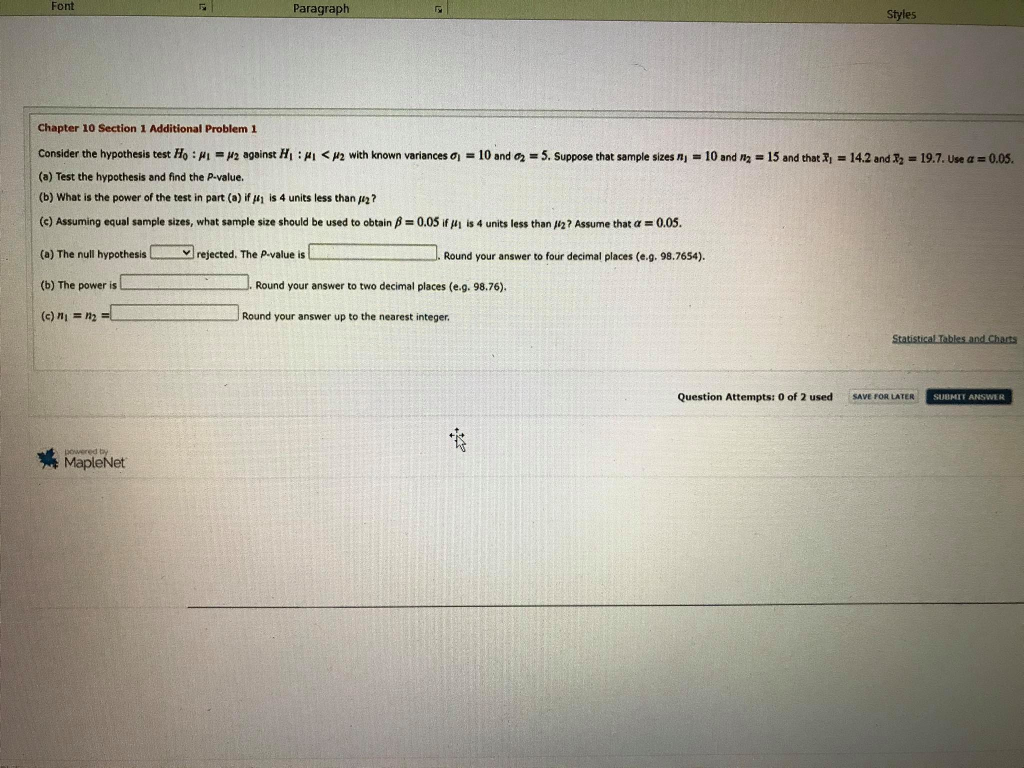Font Paragraph Styles Chapter 10 Section 1 Additional Problem 1 Consider the hypothesis test Ho : = 12 against HI : <H2 with known variances = 10 and 2 = 5. Suppose that sample sizes nj = 10 and 12 = 15 and that I = 14.2 and 72 = 19.7. Use a = 0.05. (a) Test the hypothesis and find the P-value. (b) What is the power of the test in part (a) if #1 is 4 units less than 12? (c) Assuming equal sample sizes, what sample size should be used to obtain p = 0.05 if #1 is 4 units less than 12 ? Assume that a = 0.05. (a) The null hypothesis rejected. The p-value is Round your answer to four decimal places (e.g. 98.7654). (b) The power is Round your answer to two decimal places (e.g. 98.76). (c) N = n2 =1 Round your answer up to the nearest integer. Statistical Tables and Charts Question Attempts: 0 of 2 used SAVE FOR LATER SUBMIT ANSWER powered by Maple

a)

 pop 1 pop 2 sample mean x = 14.20 19.70 std deviation σ= 10.000 5.000 sample size n= 10 15 std error σx1-x2=√(σ21/n1+σ22/n2)    = 3.416 test stat z =(x1-x2-Δo)/σx1-x2     = -1.61 p value : = 0.0537 (from excel:1*normsdist(-1.61)

a)

the null hypothesis is not rejected ; the p value is 0.0537

b)

 Hypothesized mean difference Δo       = 0 true mean difference Δ                                                          = -4 first sample standard deviation =σ1    = 10.000 second sample std deviation =σ2= 5.000 first sample size =n1 = 10.000 second sample size =n2 = 15.000 standard error=(√(σ12/n1+σ12/n1))= 3.4157 for 0.05 level and left tail critival Zα= -1.64 rejecf Ho if x<= Δo +Zα*σx or x<= -5.6017 P(Type II error) =P(Xbar>-5.602| Δ=-4)=P(Z>(-5.6017--4)/3.416)=P(Z>-0.47)=0.6808 P(Power) =1-type II error =1-0.6808=0.32

c)

 true mean difference =Δ = 4 first sample std deviation =σ1   = 10 2ndsample std deviation=σ2 = 5 for 0.05 level and left tail critical Zα= 1.645 for 0.05 level of type II error critival Zβ= 1.645 n=(Zα/2+Zβ)2(σ12+σ22)/(Δo-Δ)2 = 85
##### Add Answer of: Consider the hypothesis test H0:μ1=μ2 against H1:μ1<μ2 with known variances σ1=10 and σ2=5. Suppose that sample...
Similar Homework Help Questions
• ### X1-4.6 and consider the hypothesis test Ho: μι = μ2 against H1:让-μ2 with known variances σ1 = 9 ...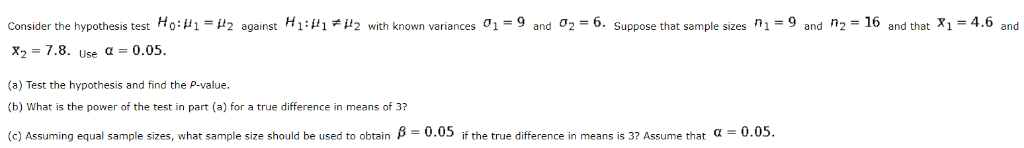x1-4.6 and consider the hypothesis test Ho: μι = μ2 against H1:让-μ2 with known variances σ1 = 9 and σ2=6 suppose that sample sizes n1= 9 and n2=16 and that X1= 4.6 and X2-7.8. Use α-0.05. (a) Test the hypothesis and find the P-value. (b) What is the power of the test in part (a) for a true difference in means of 3? (c Assuming equal sample sizes, what sample sze should be used to obtain β 0.05 the true...

• ### Consider the hypothesis test Ho : H1 = H2 against H1 : HI # Hz with...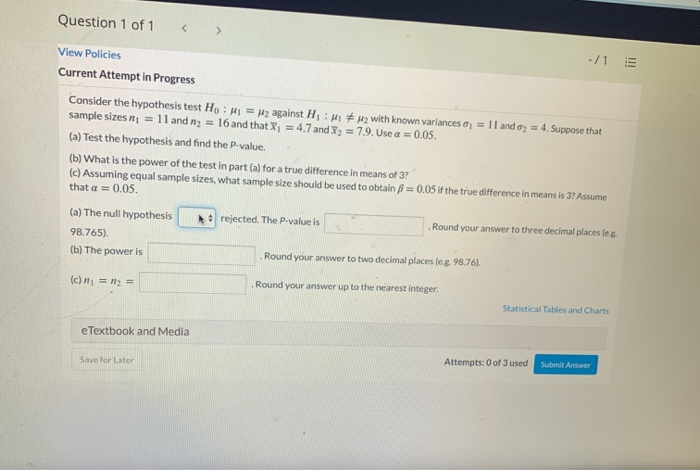Consider the hypothesis test Ho : H1 = H2 against H1 : HI # Hz with known variances oj = 1 1 and oz = 4. Suppose that sample sizes ni = 11 and n2 = 16 and that X = 4,7 and X2 = 7.9. Use a = 0.05. Question 1 of 1 < > -/1 View Policies Current Attempt in Progress Consider the hypothesis test Ho: M1 = H2 against H: MM with known variances o = 11...

• ### Consider the hypothesis test Ho: Mi = H2 against H:M1 < u2 with known variances a...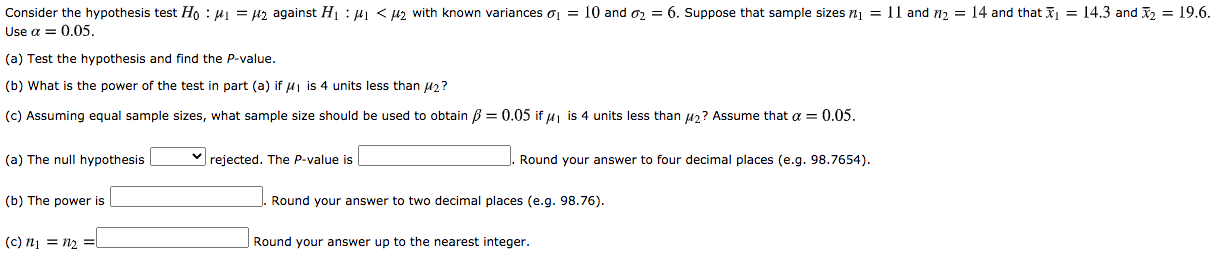Consider the hypothesis test Ho: Mi = H2 against H:M1 < u2 with known variances a = 10 and 02 = 6. Suppose that sample sizes ni = 11 and 12 = 14 and that īj = 14.3 and 12 = 19.6. Use a = 0.05. (a) Test the hypothesis and find the P-value. (b) What is the power of the test in part (a) if 1 is 4 units less than 42? (c) Assuming equal sample sizes, what sample...

• ### Chapter 10 Section 1 Additional Problem 1 Consider the hypothesis test Ho: Mi = u2 against...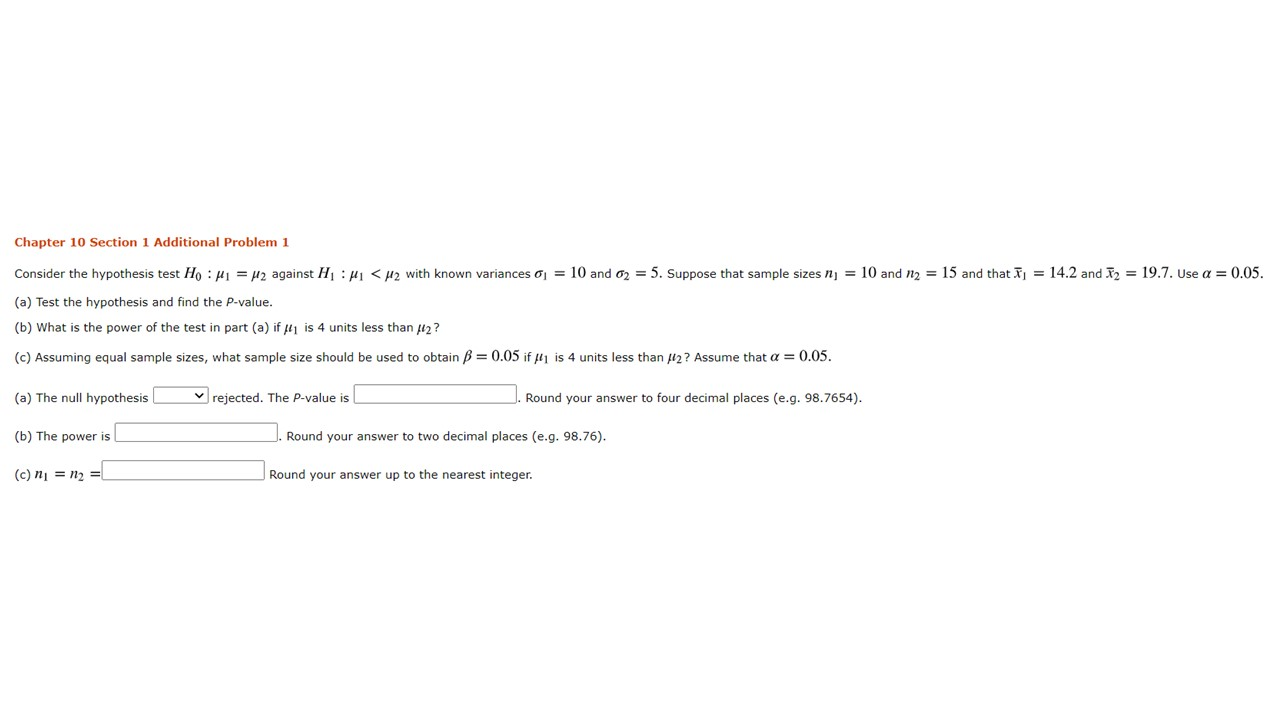Chapter 10 Section 1 Additional Problem 1 Consider the hypothesis test Ho: Mi = u2 against H:Mi < u2 with known variances ay = 10 and 62 = 5. Suppose that sample sizes nj = 10 and 12 = 15 and that j = 14.2 and I2 = 19.7. Use a = 0.05. (a) Test the hypothesis and find the P-value. (b) What is the power of the test in part (a) if ili is 4 units less than 12?...

• ### Chapter 10 Section 1 Additional Problem 1 Consider the hypothesis test Ho: M1 = H2 against...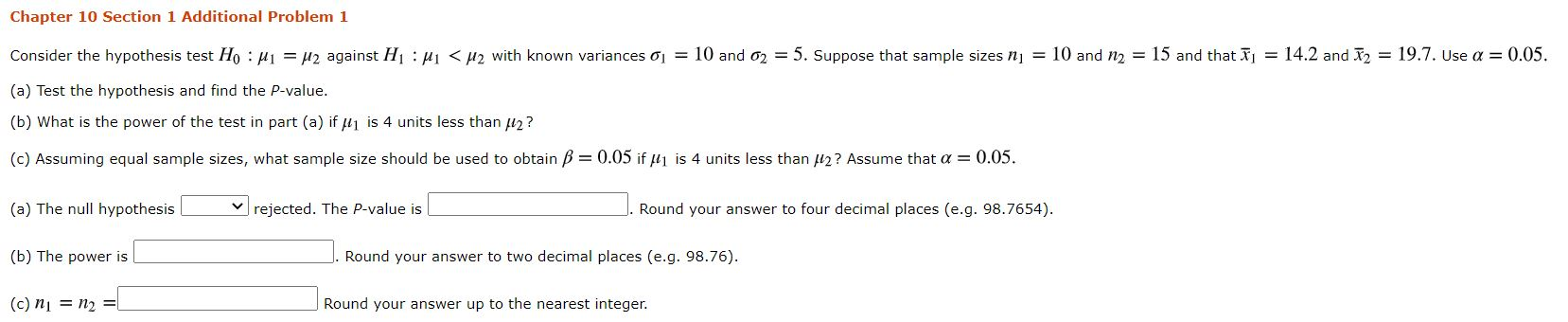Chapter 10 Section 1 Additional Problem 1 Consider the hypothesis test Ho: M1 = H2 against H:41 <H2 with known variances j = 10 and 62 = 5. Suppose that sample sizes nj = 10 and 12 = 15 and that Ij = 14.2 and 12 = 19.7. Use a = 0.05. (a) Test the hypothesis and find the P-value. (b) What is the power of the test in part (a) if u1 is 4 units less than 2? (c)...

• ### Consider the hypothesis test Ho: Mi = u2 against Hui < u2 with known variances 01...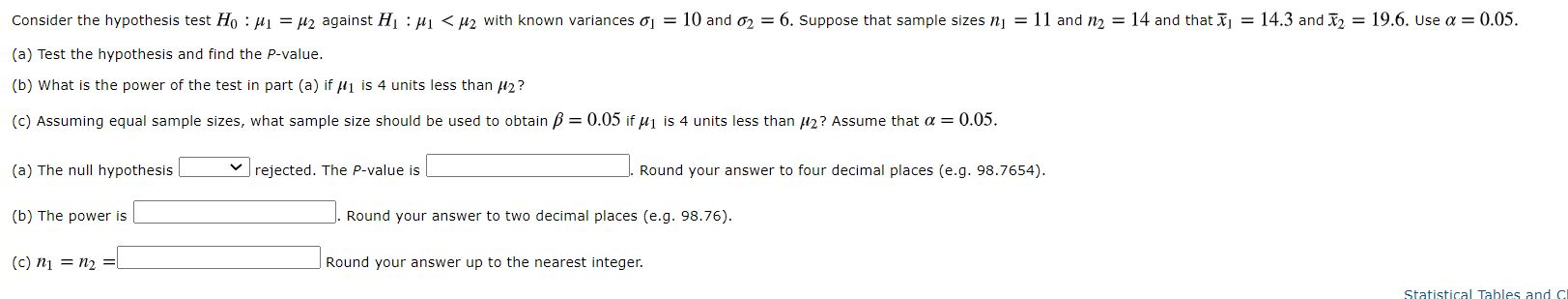Consider the hypothesis test Ho: Mi = u2 against Hui < u2 with known variances 01 = 10 and 02 = 6. Suppose that sample sizes nj = 11 and n2 = 14 and that Ij = 14.3 and I2 = 19.6. Use a = 0.05. (a) Test the hypothesis and find the P-value. (b) What is the power of the test in part (a) if fi is 4 units less than 12? (C) Assuming equal sample sizes, what sample...

• ### Consider the hypothesis test Ho: Mi = u2 against Hui <Hz with known variances j =...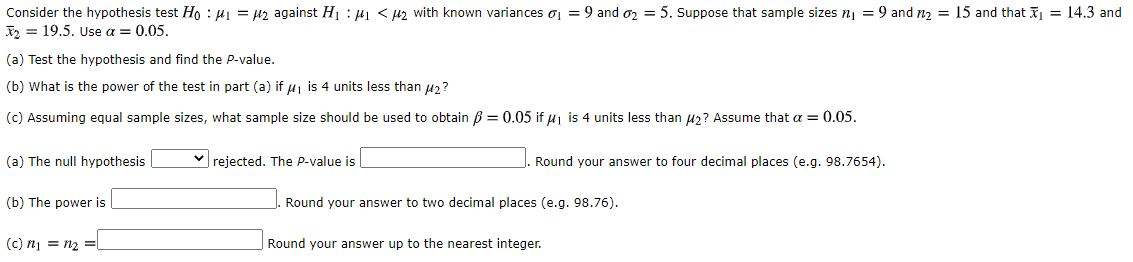Consider the hypothesis test Ho: Mi = u2 against Hui <Hz with known variances j = 9 and 02 = 5. Suppose that sample sizes n = 9 and n2 = 15 and that j = 14.3 and 12 = 19.5. Use a = 0.05. (a) Test the hypothesis and find the P-value. (b) What is the power of the test in part (a) if u is 4 units less than 12? (c) Assuming equal sample sizes, what sample size...

• ### Consider the hypothesis test Ho: Mi = U2 against HL : M1 <H2 with known variances...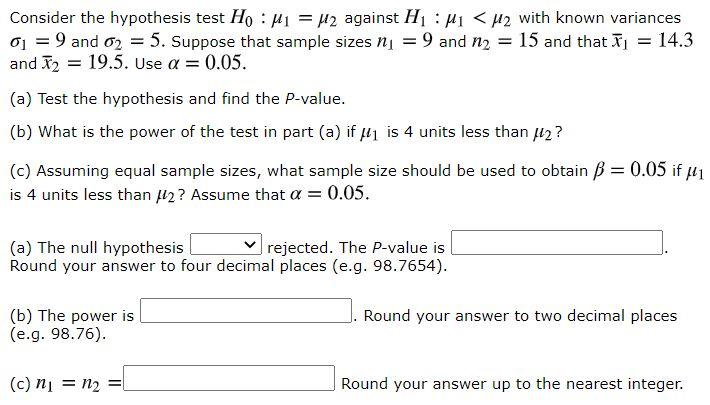Consider the hypothesis test Ho: Mi = U2 against HL : M1 <H2 with known variances a = 9 and 62 = 5. Suppose that sample sizes nj = 9 and n2 = 15 and that I = 14.3 and 12 = 19.5. Use a = 0.05. (a) Test the hypothesis and find the P-value. (b) What is the power of the test in part (a) if H1 is 4 units less than 2? (c) Assuming equal sample sizes, what...

• ### Consider the hypothesis test  H0: μ1-μ2=0 against  H1: μ1-μ2≠0 samples below: Reserve Problems Chapter 10 Section...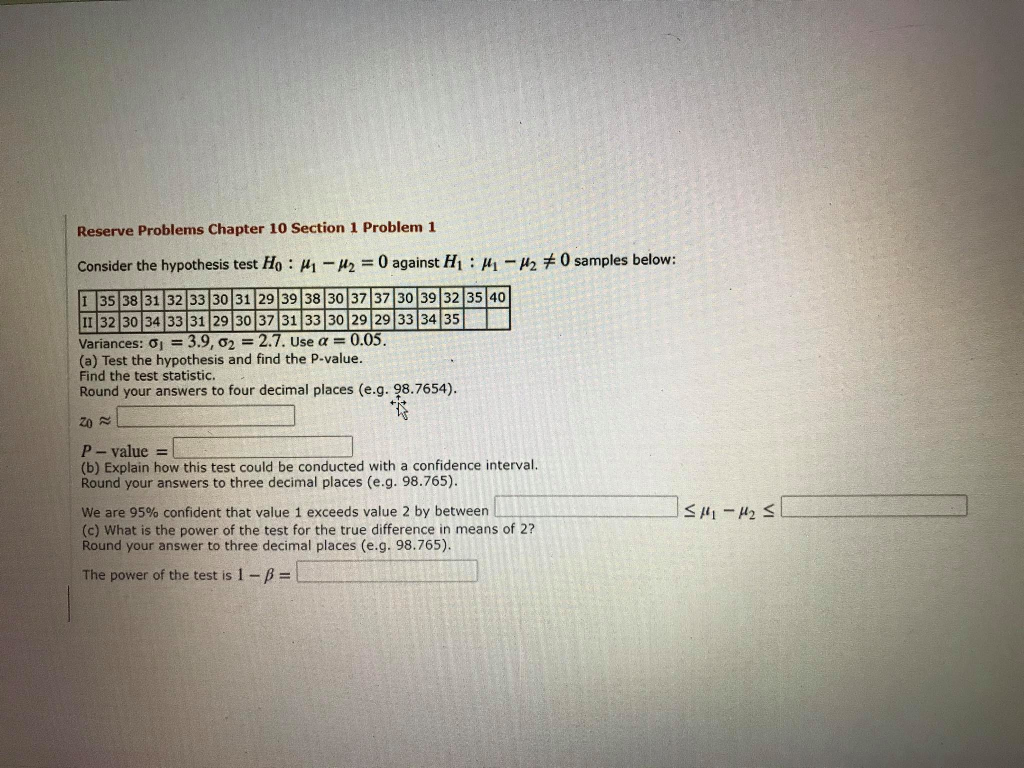Consider the hypothesis test  H0: μ1-μ2=0 against  H1: μ1-μ2≠0 samples below: Reserve Problems Chapter 10 Section 1 Problem 1 Consider the hypothesis test HO : H1 - H2 = 0 against HL : M - H270 samples below: I 35 38 31 32 33 30 31 29 39 38 30 37 37 30 39/32 3540 II |32|30|34|33|31|29 30 37 31 33 30 29 29 33 34 35 Variances: 0 = 3.9, 02 = 2.7. Use a = 0.05. (a) Test...

• ### Reserve Problems Chapter 10 Section 1 Problem 1 Consider the hypothesis test  H0: μ1-μ2=0 against  H1: ...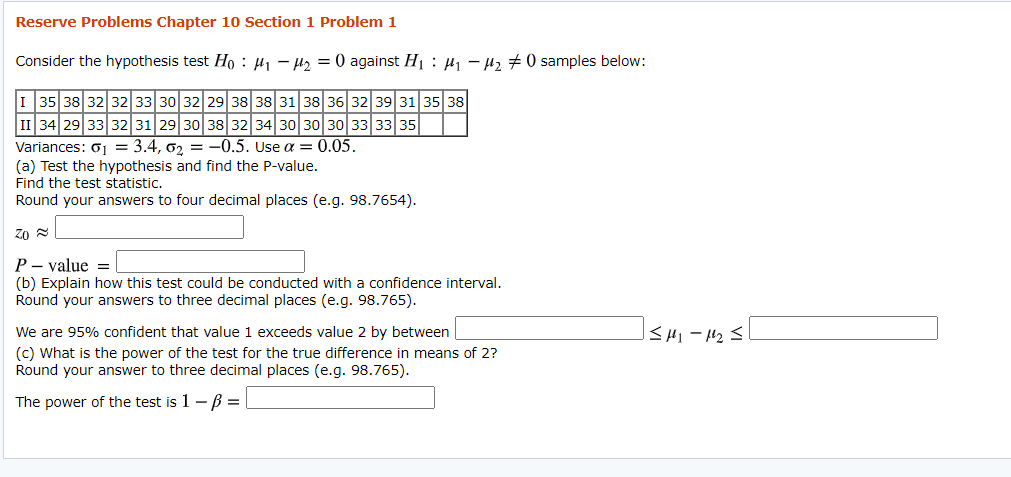Reserve Problems Chapter 10 Section 1 Problem 1 Consider the hypothesis test  H0: μ1-μ2=0 against  H1: μ1-μ2≠0 samples below: I 35 38 32 32 33 30 32 29 38 38 31 38 36 32 39 31 35 38 II 34 29 33 32 31 29 30 38 32 34 30 30 30 33 33 35 Variances: σ1=3.4, σ2=-0.5. Use α=0.05. (a) Test the hypothesis and find the P-value. Find the test statistic. Round your answers to four decimal places (e.g....

Free Homework App